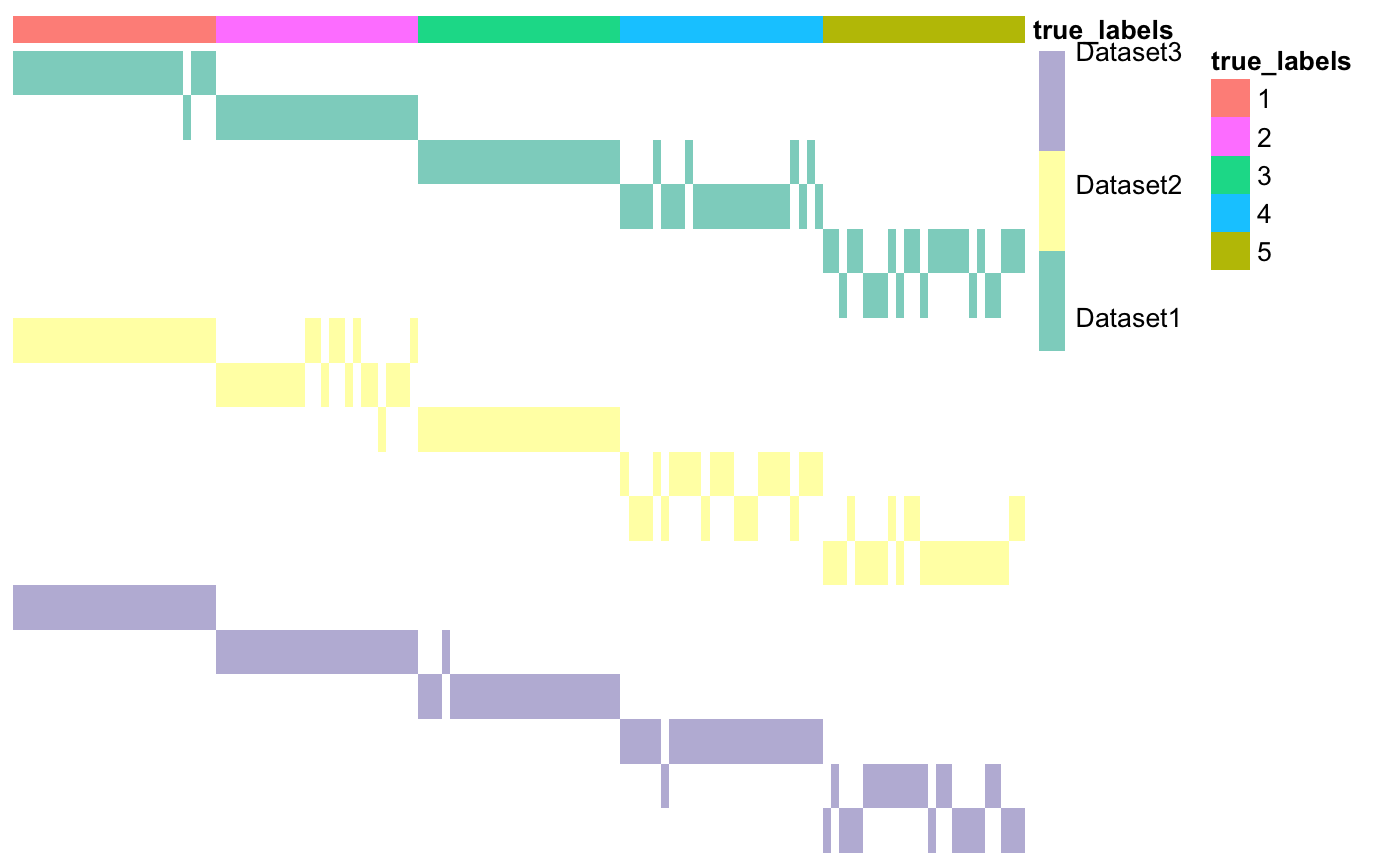This function creates a matrix of clusters, starting from a list of heterogeneous datasets.

plotMOC(
moc,
datasetIndicator,
datasetNames = NULL,
annotations = NULL,
clr = FALSE,
clc = FALSE,
savePNG = FALSE,
fileName = "moc.png",
showObsNames = FALSE,
showClusterNames = FALSE,
annotation_colors = NA
)

## Arguments

moc Matrix-Of-Clusters of size N x sumK. Vector containing integers indicating which rows correspond to some clustering of the same dataset. Vector containing the names of the datasets to which each column of labels corresponds. If NULL, datasetNames will be the same as datasetIndicator. Default is NULL. Dataframe containing annotations. Number of rows must be N. If the annotations are integers, use as.factor() for a better visual result. Cluster rows. Default is FALSE. Cluster columns. Default is FALSE. Boolean. If TRUE, plot is saved as a png file. If savePNG is TRUE, this is the string containing the name of the moc figure. Can be used to specify the folder path too. Default is "moc". The ".png" extension is automatically added to this string. Boolean. If TRUE, the plot will also include the column names (i.e. name of each observation). Default is FALSE, since there are usually too many columns. Boolean. If TRUE, plot cluster names next to corresponding row. Default is FALSE. Optional. See annotation_colors in pheatmap::pheatmap.

The Cancer Genome Atlas, 2012. Comprehensive molecular portraits of human breast tumours. Nature, 487(7407), pp.61–70.

## Examples

# Load data
data <- list()
package = "coca"), row.names = 1))
package = "coca"), row.names = 1))
package = "coca"), row.names = 1))

# Create vector of dataset names, in the same order as they appear above
datasetNames <- c("Dataset1", "Dataset2", "Dataset3")

# Build matrix of clusters
outputBuildMOC <- buildMOC(data, M = 3, K = 6, distances = "cor")

# Extract matrix of clusters and dataset indicator vector
moc <- outputBuildMOC$moc datasetIndicator <- outputBuildMOC$datasetIndicator

# Prepare annotations
annotations = annotations)